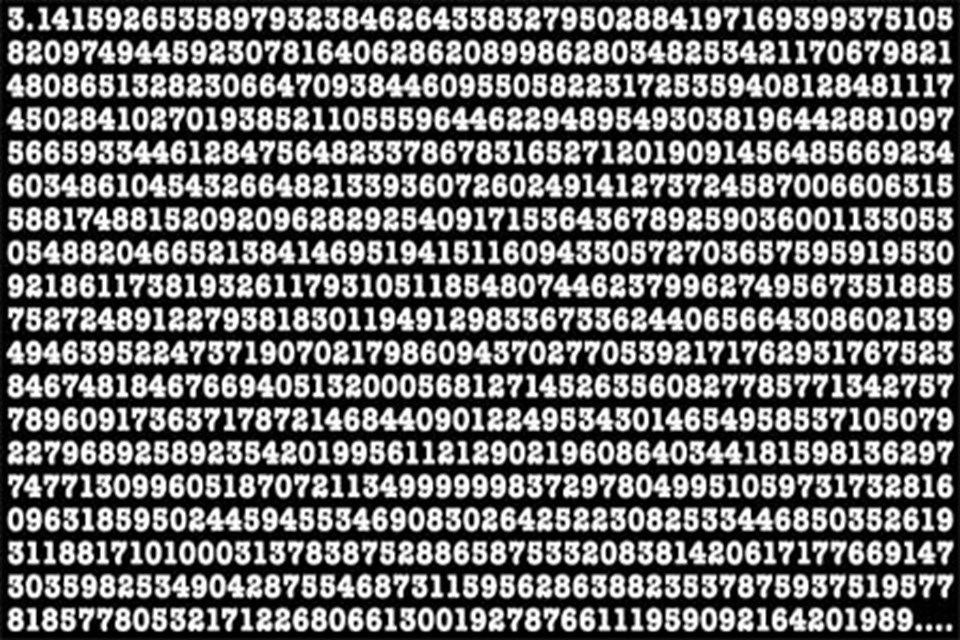# How Do We Know That Pi Is Infinite?Close-up of hand-written Pi numbers on green chalkboard. Focus on the blackboard.

How Do We Know That Pi Is Infinite?

Some have memorized the number Pi as 22 divided by 7 because they have trouble remembering the digits. Although the result of the division operation does not contain one hundred percent accuracy, it is considered 99.96% accurate, which is sufficient for practical applications.

Swiss mathematician Johann Lambert discovered in 1768 that Pi is eternal, and realized that it was impossible to calculate its value exactly. To prove this, he showed that Pi is not a rational number.

Rational numbers, when converted to decimals, usually give net results after a few digits (for example, 1/8 = 0.125). Sometimes they continue with the repetition of certain numbers (like 4/7 = 0.571428571…)

A different situation applies to the Pi, on the other hand. The sequence of digits after the comma is never the same. It is constantly changing, it goes on and on without being repeated in any way.

In fact, the number pi has been used since ancient times (2000 BC). Because it is closely related to a very commonly used geometric object such as a circle.

As we all know, the number pi is the length of the circle divided by its diameter. Although the dimensions of the circle change, this ratio does not change at all. That’s why it’s one of those special numbers called mathematical constants.

In August, 62.8 trillion digits of Pi were calculated.How Do We Know That Pi Is Infinite? Some have memorized the number Pi as 22 divided by 7 because they have trouble remembering the digits. Although the result of the division operation does not contain one hundred percent accuracy, it is considered 99.96% accurate, which is sufficient for practical applications.

WHAT DOES THE SYMBOL MEAN?
The symbol Pi is the 16th letter of the Greek alphabet.

WHAT IS PIPHILLOGY?
It is the name given to all the methods used to memorize the number pi.

`Source: https://www.ntv.com.tr/& https://popsci.com.tr/`